# Grid Side Inverter Control for a Grid Connected Synchronous Generator Based Wind Turbine Experimental Emulator

Grid Side Inverter Control for a Grid Connected Synchronous Generator Based Wind Turbine Experimental Emulator

Dekali ZouheyrBaghli Lotfi Lubin Thierry Boumediene Abdelmadjid

Laboratoire d'Automatique de Tlemcen, Université de Tlemcen, Tlemcen 13000, Algeria

GREEN, EA 4366, Université de Lorraine, Vandoeuvre-lès-Nancy F-54500, France

Corresponding Author Email:
zouheyr.dekali@univ-tlemcen.dz
Page:
1-7
|
DOI:
https://doi.org/10.18280/ejee.230101
8 October 2019
|
Accepted:
14 December 2020
|
Published:
28 February 2021
| Citation

OPEN ACCESS

Abstract:

This paper describes the real time implementation and control of a wind energy conversion chain emulator based on a synchronous generator (SG) using a full-scale power converter configuration. The proposed structure consists of the mechanical coupling of two 1.5 kW machines, a DC motor which emulates the static-dynamic behaviors of a three-blade wind turbine with a horizontal axis including an ideal gearbox, and a synchronous generator that ensures the electromechanical conversion and manages the different operating modes. The aim of the first part in this work is the design and the implementation of the control of the grid side converter in order to control the flow of the produced/consumed active and reactive power (PGSC / QGSC) in both directions between the generator and the grid. An improved experimental grid voltage vector-orientation control algorithm (VOC) is investigated and applied to the grid inverter to control the GSC powers independently and instantly. The control algorithms are implanted in C, using dSPACE DS1104 control board to drive the 6-IGBT’s inverter. The experimental results validate the effectiveness of the proposed control scheme of the GSC.

Keywords:

wind energy conversion system, synchronous generator, grid side converter, DC bus voltage, dSPACE DS1104

1. Introduction

Research and teaching interests in renewable energies and generation systems are growing. The projects at the laboratory concerns the design, implantation and intelligent management of an energy production system which is composed of two wind turbine emulator (double fed induction generator (DFIG) and synchronous generator (SG)), and a photovoltaic system. The results of this work will be used for training, PhD researchers and also for national needs in the field of renewable energies [1, 2].

This work is a continuation of a previous theoretical and practical research in the field of wind energy conversion system, that falls with the lab framework project that aims to the real-time design, implementation and intelligent management of smart distributed renewable energy micro-power plants [3, 4]. The project develops four types of micro-power plants: 1.5 kW Double Fed Induction Generator Based Wind Turbine Emulator, 1.5 kW Synchronous Generator Based Wind Turbine Emulator, Photovoltaic system with Grid Tie Inverters and finally, a 250 W Photovoltaic micro-power plant connected through a self-made single phase Grid Tie Inverter [2, 5, 6].

Furthermore, as first part, our previous works carried out in papers [1, 2], focuses on the experimental implementation of a grid connected variable-speed concept based wind power system, with partly rated power converters. The emulator is equipped with the DFIG configuration. The aim of this research is to build a low-cost experimental bench for the emulation of a wind turbine's conversion chain, with the use of a controlled DC armature motor for the aerodynamic drive emulation. In both hypo synchronous and hypersynchronous modes. This device handles multiple scenarios and operating points, and allow to evaluate numerous control laws (linear / non-linear) for the generator power control. The power HIL was tested successfully thanks to the DFIG PI linear control law. However, a nonlinear control law would be more flexible in order to improve the quality of the control and reduce the operating constraints.

The second prototype consists of a 1.5 kW photovoltaic industrial micro power plant, six 250 W PV panels connected to three Grid Tie Inverters (GTI), with power line communication Unit (ECU). A Raspberry Pi3 (RPi3) reads the data of the generated power from the web server of the ECU and send them to the Firebase Database (DB). We develop strategies to control the micro power plants in a way that each one produces the exact quantity of power in real-time to follow the consumption variation and the weather changes.

The third system is a 250 W photovoltaic self-made micro power plant and is strongly related to the previous part. It implements a single-phase GTI. The study outlines indirect active and reactive power injection using a zero-crossing detection (ZCD) method to master the synchronization of the generated voltage from the inverter and the power grid [5, 6].

The successful implementation of the contribution established in this paper will allow the extraction of emulated electrical wind energy in order to inject it into the common system grid according to the needs determined by the intelligent management of the micropower plant system. On the other hand, the control technique of the grid side inverter can be added to the DFIG setup instead of the all-diode rectifier.

Nowadays, the synchronous generator based wind turbine system is one of the most popular configurations that use the concept of full-scale power electronic converter. This is due to its large advantage in reducing sensitivity against the grid voltage disturbance, moreover, the good operating under variable speeds thanks to the full control of the generator, consequently, achieve the maximum efficiency, with a low synchronous speed thanks to a high poles number, add to that, a protection of the generator by isolating it from the grid, unlike the DFIG configuration [7-9].

Many authors have discussed the fully rated power converters based variable speed wind turbine system. Hansen and Michalke  discussed the modeling and control of a full-scale back-to-back converter wind turbine with multi-pole permanent magnet synchronous generator PMSG. They focus on the grid support capability in uninterrupted operation during grid faults. The paper  provides a detailed and robust model for a back-to-back converter of a multi-pole PMSG wind turbine. A damping controller is implemented in order to actively damp the torsional oscillations in the drive-train to absorb the system stress. The active and reactive power is controlled thank to the grid-side converter, while the machine side converter keeps the stator voltage and the DC bus voltage constant. In the paper , the authors use the hybrid adaptive control method for adjusting the DC link voltage in the weak-grid mode for a connected PMSG. The control algorithm is evaluated in both simulation and experimental environments. Berkani et al.  investigated an improvement of the control of the DC link voltage of a dual star synchronous machine. The authors propose the sliding mode approach to drive the gride side inverter, while keeping a conventional PI controller for the machine side inverter drive. The work  investigates the experimental implementation of a predictive control model algorithm for a connected two-level voltage source converter. The paper details clearly the four steps of the proposed technique, the authors focus on the performance and the efficiency. The control board DS1104 used is the same as in our experimental setup.

Our paper is organized as follows: Section 2 gives the detailed description of the overall proposed structure of this wind energy emulator with a presentation of all the experimental bench elements (machines, power electronic converters, measurements...). In section 3, we present the GSC modeling, including the (Rf, Lf) filter and the DC link connection, with its control algorithm. In section 4, the experimental results show the control of the active and reactive power transfer between the grid and GSC. Finally, section 5 gives some conclusions.

2. Hardware Description of the Wind Turbine Emulator

Figure 1 shows the complete diagram of our experimental bench, which consists in a wind turbine emulator based on a grid connected synchronous machine. A 1.5 kW DC motor is mechanically coupled to the SG shaft in order to simulate the wind turbine drive, hence, to get the needed aerodynamic torque. The DC motor is powered through a chopper, and its power comes from an independent DC power supply source. The SG is driven by a 6-IGBT’s inverter, the machine side converter (MSC) that imposes the current references for reaching the desired SG active and reactive power through vector control [1, 2, 15].

The machine side converter (MSC) is powered by a second 6-IGBT inverter which is called grid side converter (GSC) through a DC link contains a filtering capacitor. The GSC main role is the control of direction and the power consumed or injected into the grid by the acting on the phases currents (Iqf, Idf). An autotransformer is used for testing and to adapt the grid voltage to the system.

We focus in this paper on the control of the GSC, for this, we use a dSPACE DS1104 control board to generates 6 PWM signals in order to control the 3-legs IGBT’s grid side inverter. The same board will be used to control the MSC and the DC chopper, by changing the internal firmware of its slave DSP and with proper programming of its master controller (PowerPC).

We use the following ADC measures: The DC bus voltage (VDC), 2 GSC phase voltages (Vsa, Vsb), 2 GSC phase currents (Ifa, Ifb). For the MSC, we will need (Imsa, Imsb) and for the DC motor torque control (wind emulator), we will measure its current (IDCM). We use the LEM LA55 Hall effect sensors for the ADC current measurements and the LEM LV25-P Hall effect sensors for the voltages. Proper active filtering and signal conditioning is added before to the ADC stage.

The Control Desk™ running on the DS1104 host PC allows the visualization and interaction with the system variables in real time. The lab set up is shown in Figure 13 (See Appendix), while the hardware components are exposed in Table 1 (See Appendix). The nominal parameters of the experimental bench are given in Table 2 (See Appendix).

## 1.pngFigure 1. Experimental structure of the wind turbine emulator based on a SG

3. GSC Modelling and Control

GSC is responsible for the DC bus voltage adjustment and the power flow from and to the grid. As a first step in the implementation of this emulator, we start by testing only the grid side inverter without the entire emulator, based on that, we introduce the simplified model of the filter-inverter-DC bus assembly in the next description .

3.1 Grid-Filter-Inverter modeling

According to Kirchhoff law applied to the circuit of Figure 2, we express the phase voltages of the GSC, the grid and the filter in the abc reference framework. We consider a receptor convention [16, 17].

## 2.png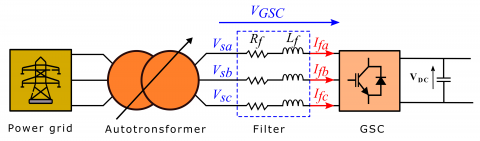Figure 2. Representation of the GSC inverter, the filter and the grid

The phase voltages between the GSC and the grid can be expressed in the stationary abc reference frame as follows :

$V_{G S C_{a b c}}=R_{f} I_{f_{a b c}}+L_{f} \frac{d I_{f_{a b c}}}{d t}+V_{S_{a b c}}$     (1)

In order to apply the vector control principle, we express the previous model in the dq reference frame. The GSC voltages (Vdf, Vqf) are given by [8, 19]:

$V_{d f}=R_{f} I_{d f}+L_{f} \frac{d I_{d f}}{d t}-\omega_{S} L_{f} I_{q f}+V_{d s}$     (2)

$V_{q f}=R_{f} I_{q f}+L_{f} \frac{d I_{q f}}{d t}+\omega_{s} L_{f} I_{d f}+V_{q s}$     (3)

The active power and the reactive power of the GSC in the dq reference frame are described by :

$P_{G S C}=V_{d s} I_{d f}+V_{q s} I_{q f}$    (4)

$Q_{G S C}=V_{q s} I_{d f}-V_{d s} I_{q f}$     (5)

3.2 GSC control

Figure 3 shows the overall structure of the GSC control, the main objective is to control the voltage in the DC link, and the active and reactive power (PGSC, QGSC) delivered to the grid by acting on the phases current through two current control loops . The control of the active power is linked to the control of the DC bus voltage .

To control the GSC powers PGSC(Iqf), QGSC(Idf) we apply a grid voltage vector-orientation control (VOC). Thus, the grid voltage vector Vs is hold on the q-axis (Vqs=Us, Vds=0). We give the expression of the GSC powers according to the GSC currents as follows .

$P_{G S C}=U_{s} I_{q f}$      (6)

$Q_{G S C}=U_{s} I_{d f}$     (7)

## 3.pngFigure 3. Schematic diagram of the GSC control

The decoupled model obtained allows controlling the GSC powers independently. For the GSC active power PGSC control, we act on GSC quadrature current Iqf and we act on the GSC direct current Idf for GSC reactive power control . The GSC voltages references, which are the outputs of the current controllers, are given by Amrane et al. :

$V_{d f}=R_{f} I_{d f}+L f \frac{d I_{d f}}{d t}-\omega_{s} L_{f} I_{q f}$     (8)

$V_{q f}=R_{f} I_{q f}+L f \frac{d I_{q f}}{d t}+\omega_{s} L_{f} I_{d f}+U_{s}$      (9)

Figure 4 shows the detailed structure of the GSC control. The error signal between the measured value (Iqf, Idf) and the reference value (Iqf*, Idf*) are fed in a PI controller. The power reference steps (QGSCor Idf*), (PGSC or Iqf*) are imposed manually through the Control Desk interface. In typical control systems, the reactive power reference is set to zero to perform the operating at a unity power factor. The introduction of the decoupling block with the correct calculation of the controller parameters ensures great performances of the power control system .

## 4.png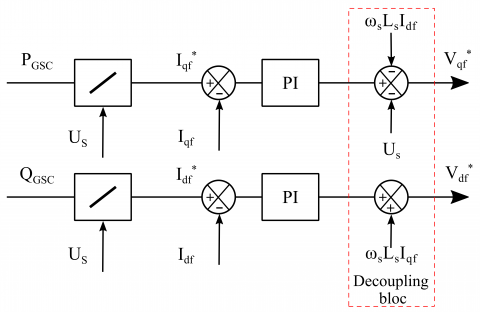Figure 4. Detailed control loop diagram of the GSC currents schematic diagram of the GSC control

3.3 DC Bus modeling and control

The power flowed in the DC bus as shown in Figure 5 are thus expressed by [18, 25]:

$P_{C a p}=P_{G S C}-P_{M S C}$     (10)

where, PCap is the power absorbed by the capacitor, PGSC the power delivered by the GSC, and PMSC the power absorbed by the MSC . Negative values mean that the devices are generating power .

## 5.pngPcapcan be expressed by its linearized expression:

$P_{C a p}=V_{D C} C \frac{d V_{D C}}{d t}$    (11)

From Eq. (10) and Eq. (11), the DC bus voltage expression is given as:

$\frac{d V_{D C}}{d t}=\frac{1}{C} \frac{P_{G S C}-P_{M S C}}{V_{D C}}$     (12)

Figure 6 shows the block diagram of the DC bus voltage control loop, the role of this control loop is keeping the DC link voltage constant and assuring that all the power coming from the MSC is instantaneously transferred to the grid through the GSC [20, 28].

## 6.png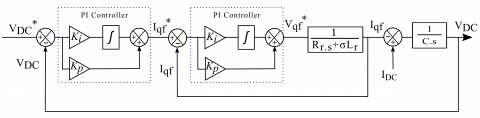Figure 6. DC bus voltage control loop

4. Experiment Results and Interpretation

Building the experimental bench is done step by step. We first evaluate the performances of the GSC control using PI controllers. Further part will be the wind turbine emulator consisting of the SG controlled by the MSC and driven by the DC motor. The real-time reference changes are made thanks to the Control Desk™ software running on the DS1104 host PC. The interface allows the visualization of the system variables and interaction in real-time. As well as the capture of data of the estimated currents, voltages and powers. Therefore, we present two tests with the Grid Side Converter control.

4.1 First test

At t = 0s, we apply a disturbance consisting in a sudden power load on the DC bus in order to show the control of the DC voltage at a reference of VDC* = 100 V. The reactive power reference is scheduled at QGSC* = 0 VAr for an unity power factor. The measured DC link voltage VDC perfectly follows its reference VDC* after a brief transient (Figure 7).

## 7.png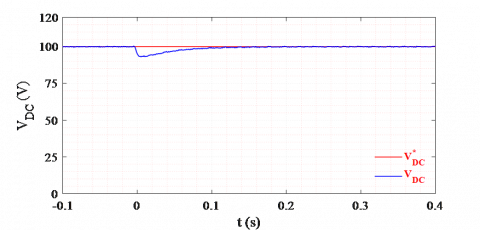Figure 7. DC bus voltage control

The disturbance is rejected thanks to the injection by the control system of the right amount of active power PGSC by the GSC. The DC bus link absorbs an active power from the grid. The VDC control loop adjusts the reference Iqf* around 4 A, in order to impose this active power. The reactive power QGSC remains constant around 0 VAr with non-important oscillations during all the test, therefore the GSC direct current reference is Idf* = 0 A. The estimated active PGSC and reactive power QGSC fluctuations also relays on the Us (Vqs) measurement, which is not so perfect, consequently the estimated active power is around -180 W with oscillations ±20 W (11%) max in steady state (Figures 8a and 8b). The measured GSC currents (Iqf, Idf) follow their references (Iqf*, Idf*) correctly thanks to the high performance of the PI controllers (Figures 8c and 8d).

## 8.png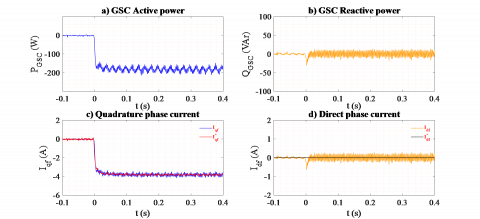Figure 8. GSC Power control

## 9.pngFigure 9. a) GSC voltages, b) Phase voltages

Figure 9a shows the necessary GSC voltages references (Vdf*, Vqf*) needed to track the desired currents (Iqf, Idf) and consequently, the power references. The grid voltage, after the autotransformer, Us (Vqs) (Vqs= 0) is measured around 50 V. We used a low voltage for the test to operate with a DC voltage around 100 V. The sinusoidal voltages of the phases after the autotransformer have an amplitude of 40 V (Figure 9b).

4.2 Second test

In the second test, we still apply the reference of VDC* = 100 V, with a step on the reactive power QGSC* from 200 VAr to - 200 VAr. The measured DC bus voltage VDC track its reference VDC* of 100 V without almost any disturbance (Figure 10).

## 10.png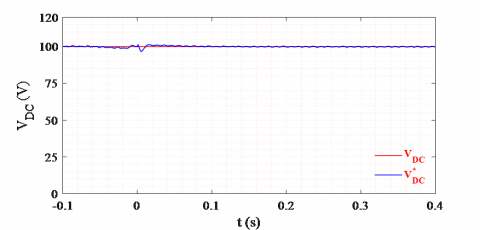Figure 10. DC bus voltage control

The reactive power follows its reference step correctly from 200 VAr to -200 VAr thanks to the direct current control (Figure 11b). Figure 11c and 11d present the same dynamic performances of the GSC current control as the previous experiment. The curve of the controlled GSC direct current Idf shows a good tracking accuracy, with an -8 A step. The direct current value will be stabilized around -4 A with negligible oscillations in steady state. Iqf response shows a single spike of 2 A in transient state, in order to reject any VDC disturbances, then remains at around -4 A, delivering an active power of -200 W to the grid (Figure 11a).

## 11.png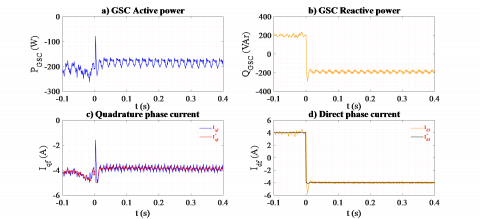Figure 11. GSC Power control

## 12.png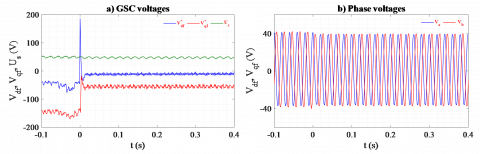Figure 12. a) GSC Voltages, b) Phase voltages

Figure 12a shows the necessary GSC voltage references that are outputted by the (Iqf, Idf) PI current controllers with significant peaks at the transient state, especially Vdf* voltage reference, that is affected by the large reference change in QGSC* (from 200 VAr to -200 VAr at t=0s). The grid voltage Us (Vqs) is always constant at 50 V, (Figure 12b).

5. Conclusions

The study discussed in this work is part of recent research on the introduction of intelligent micro-power plants in micro-grids. A 1. 5 kW synchronous generator-based wind power emulator is built according to the variable speed concept with full power electronic converter configuration. The purpose of the current work aims with the design and the control implementation of the VOC control technique, in order to drive the 6-IGBT's grid inverter. The technique allows the control of the delivered active and reactive power from and into the grid, in an instantaneous and independent way.

The grid active and reactive power are adjusted by acting on the dq grid currents, via both current control loops using PI controllers. They drive the current with enough precision and high performances. The future work is to complete this experimental setup by the implantation of the DC motor control to elaborate complex wind speed profiles in parallel with the implementation of the machine side converter (MSC) control to drive the SG. This will deliver the optimum extracted power, using MPPT, to the grid.

Acknowledgment

This work is supported by the Direction Generale de la Recherche Scientifique et du Developpement Technologique (DG RSDT).

Nomenclature
 VGSCabc a, b and c phase voltages of the GSC IGSCabc a, b and c phase currents of the GSC VSabc a, b and c phase voltages of the grid PGSC GSC active power QGSC GSC reactive power PMSC MSC active power QMSC MSC reactive power PCap DC Link active power C capacitor Vdqf direct and quadrature phase voltage Idqf direct and quadrature phase current Us phase voltage VDC DC Bus voltage Lf inductance of filter Rf resistor of filter
Appendix

Table 1. WTE hardware component

 Component Name 1 PC 2 DCM 3 SG 4 Inverter (GSC) 5 Inverter (MSC) 6 Autotransformer 7 DS1104 interface 8 Filter 9 Sensors

Table 2. Experimental bench nominal parameters

 Settings WT DCM SG Nominal power 3 kW 3 kW 3 kW Nominal speed - 1,500 rpm 1,500 rpm Nominal voltage - 220 V 230 / 400 V

## 13.png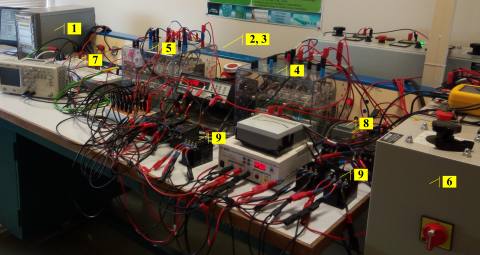Figure 13. Experimental laboratory setup

References

 Dekali, Z., Baghli, L., Boumediene, A., Djemai, M. (2018). Control of a grid connected DFIG based wind turbine emulator. 5th International Symposium on Environment-Friendly Energies and Applications, Rome, Italy, pp. 1-6. https://doi.org/10.1109/EFEA.2018.8617049

 Zouheyr, D., Baghli, L., Boumediene, A. (2019). Indirect power control for a grid connected double fed induction generator based wind turbine emulator. International Conference on Advanced Electrical Engineering, Algiers, pp. 1-6. https://doi.org/10.1109/ICAEE47123.2019.9014778

 Terfa, H., Baghli, L., Bhandari, R. (2020). Distributed renewable energy micro-power plants: A solution for new and existing power grids over Africa. 4th SEE SDEWES conference, Sarajevo, pp. 1-13.

 Terfa, H., Baghli, L., Bhandari, R. (2018). Real time simulations of power systems in transient stability with power system stabilizers. PAUWES Research-2-Practice Forum 2018 - PAUWES CoP, Tlemcen, pp. 1-6.

 Merah, M., Baghli, L., Boumediene, A. (2018). Simulation and comparison between conventional and interleaved buck-boost converter for grid-connected PV system. PAUWES Research-2-Practice Forum 2018 - PAUWES CoP, Tlemcen, pp. 1-6. https://doi.org/10.2139/ssrn.3210539

 Merah, M., Baghli, L., Boumediene, A. (2019). Prototyping of photovoltaic grid-tie inverter with active and reactive power injection. International Conference on Advanced Electrical Engineering, Algiers, pp. 1-6. https://doi.org/10.1109/ICAEE47123.2019.9014764

 Xu, D.H., Blaabjerg, F., Chen, W.J., Zhu, N. (2018). Advanced Control of Doubly Fed Induction Generator for Wind Power Systems. Wiley-IEEE Press.

 Zhang, Y.Z., Liu, H.Y., Mantooth, H.A. (2016). Control strategy of high power converters with synchronous generator characteristics for PMSG-based wind power application. IEEE Applied Power Electronics Conference and Exposition, Long Beach, CA, pp. 3180-3184. https://doi.org/10.1109/APEC.2016.7468319

 Llano, D., McMahon, R., Tatlow, M. (2014). Control algorithms for permanent magnet generators evaluated on a wind turbine emulator test-rig. 7th IET International Conference on Power Electronics, Machines and Drives, Manchester, UK, pp. 1-7. https://doi.org/10.1049/cp.2014.0304

 Hansen, A.D., Michalke, G. (2009). Multi-pole permanent magnet synchronous generator wind turbines’ grid support capability in uninterrupted operation during grid faults. IET Renewable Power Generation, 3(3): 333-348. https://doi.org/10.1049/iet-rpg.2008.0055

 Hansen, A.D., Michalke, G. (2008). Modelling and control of variable-speed multi-pole permanent magnet synchronous generator wind turbine. Wind Energy, 11(5): 537-554. https://doi.org/10.1002/we.278

 Yuan, X.B., Wang, F., Boroyevich, D., Li, Y.D., Burgos, R. (2009). DC-link voltage control of a full power converter for wind generator operating in weak-grid systems. IEEE Transactions on Power Electronics, 24(9): 2178-2192. https://doi.org/10.1109/TPEL.2009.2022082

 Berkani, A., Negadi, K., Allaoui, T., Marignetti, F. (2019). Sliding mode control of wind energy conversion system using dual star synchronous machine and three level converter. Tecnica Italiana-Italian Journal of Engineering Science, 63(2-4): 243-250. https://doi.org/10.18280/ti-ijes.632-418

 Berkani, A., Negadi, K., Safa, A., Marignetti, F. (2020). Design and practical investigation of a model predictive control for power grid converter. Tecnica Italiana-Italian Journal of Engineering Science, 64(2-4): 354-360. https://doi.org/10.18280/ti-ijes.642-434

 Zouheyr, D., Baghli, L., Boumediene, A. (2019). Experimental emulation of a small wind turbine under operating modes using DC motor. 4th International Conference on Power Electronics and their Applications, Elazig, Turkey, pp. 1-5. https://doi.org/10.1109/ICPEA1.2019.8911194

 Song, S.H., Kang, S.I., Hahm, N.K. (2003). Implementation and control of grid connected AC-DC-AC power converter for variable speed wind energy conversion system. 18th Annual Applied Power Electronics Conference, Miami Beach, FL, pp. 154-158. https://doi.org/10.1109/APEC.2003.1179207

 Li, S.H., Haskew, T.A., Swatloski, R.P., Gathings, W. (2012). Optimal and direct-current vector control of direct-driven PMSG wind turbines. IEEE Transactions on Power Electronics, 27(5): 2325-2337. https://doi.org/10.1109/TPEL.2011.2174254

 Phankong, N., Manmai, S., Bhumkittipich, K., Nakawiwat, P. (2013). Modeling of grid-connected with permanent magnet synchronous generator (PMSG) using voltage vector control. Energy Procedia, 34: 262-272. https://doi.org/10.1016/j.egypro.2013.06.754

 Rahimi, M. (2017). Modeling, control and stability analysis of grid connected PMSG based wind turbine assisted with diode rectifier and boost converter. International Journal of Electrical Power & Energy Systems, 93: 84-96. https://doi.org/10.1016/j.ijepes.2017.05.019

 Aboudrar, I., El Hani, S., Mediouni, H., Aghmadi, A. (2018). Modeling and robust control of a grid connected direct driven PMSG wind turbine by ADRC. Advances in Electrical and Electronic Engineering, 16(4): 402-413. https://doi.org/10.15598/aeee.v16i4.2952

 Youness, E.M., Aziz, D., Abdelaziz, E.G., Jamal, B., Najib, E.O., Othmane, Z., Khalid, M., Bossoufi, B. (2019). Implementation and validation of backstepping control for PMSG wind turbine using DSPACE controller board. Energy Reports, 5: 807-821. https://doi.org/10.1016/j.egyr.2019.06.015

 Xu, D., Blaabjerg, F., Chen, W., Zhu, N. (2018). Control of DFIG Power Converters: Advanced Control of Doubly Fed Induction Generator for Wind Power Systems. Wiley-IEEE Press.

 Fayssal, A., Chaiba, A., Babes, B.E., Mekhilef, S. (2016). Design and implementation of high performance field oriented control for grid-connected doubly fed induction generator via hysteresis rotor current controller. Revue Roumaine des Sciences Techniques - Serie Électrotechnique et Énergétique, 61(4): 319-324.

 Alaboudy, A.H.K., Daoud, A.A., Desouky, S.S., Salem, A.A. (2013). Converter controls and flicker study of PMSG-based grid connected wind turbines. Ain Shams Engineering Journal, 4(1): 75-91. https://doi.org/10.1016/j.asej.2012.06.002

 Van, T.L., Ngyen, T.D., Tran, T.T., Nguyen, H.D. (2015). Advanced control strategy of back-to-back PWM converters in PMSG wind power system. Advances in Electrical and Electronic Engineering, 13(2): 81-95. https://doi.org/10.15598/aeee.v13i2.1161

 Zhou, D., Blaabjerg, F. (2017). Bandwidth oriented proportional-integral controller design for back-to-back power converters in DFIG wind turbine system. IET Renewable Power Generation, 11(7): 941-951. https://doi.org/10.1049/iet-rpg.2016.0760

 Ismail, G., Mohamed Toufik, B., Said, B. (2018). Real time implementation of feedback linearization control based three phase shunt active power filter. European Journal of Electrical Engineering, 20(4): 517-532. https://doi.org/10.3166/ejee.20.517-532

 Chau, T.K., Yu, S.S., Fernando, T.L., Lu, H.H.C., Small, M. (2018). A novel control strategy of DFIG wind turbines in complex power systems for enhancement of primary frequency response and LFOD. IEEE Transactions on Power Systems, 33(2): 1811-1823. https://doi.org/10.1109/TPWRS.2017.2726160